Practice - Sequence of Transformations Terms of Use    Contact Person: Donna Roberts1.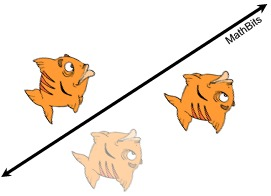Does this fish
illustration depict
a glide reflection?

 yes no

2.

The point (3,−2) is rotated 90º about the origin and then dilated by a scale factor of 4. What are the coordinates of the resulting image?

Choose:
 (-12,8) (12,-8) (8,12) (-8,-12)

3.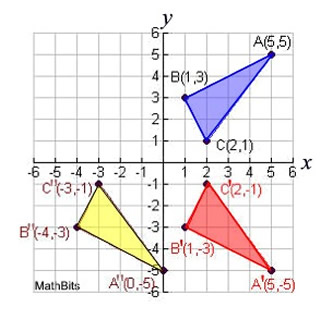Is ΔA''B''C''
a glide reflection
of Δ ABC?

 yes no

4.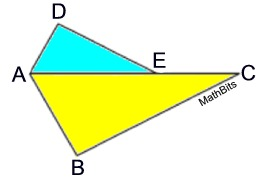ΔADE is the image of ΔABC after a reflection over the line through points A and C, followed by a dilation of scale factor ½ centered at point A.
Which statement must be true?

Choose:
 m∠BAC = m∠AED m∠ABC = m∠ADE m∠DAE=½ • m∠BAC m∠ACB = ½ • m∠DAB

5.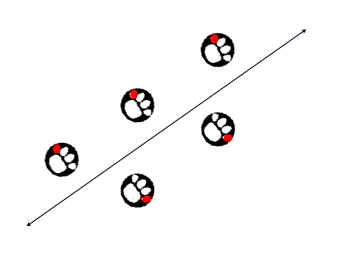Does this paw print illustration depict a glide reflection?

 yes no

6.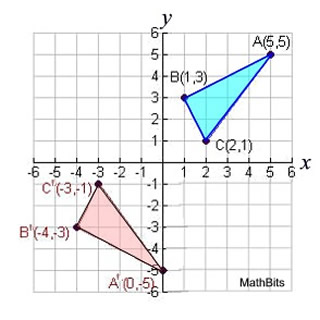ΔABC is transformed to ΔA'B'C'.
Which transformation sequence does NOT depict this change?

Choose:
 reflection over x-axis, then translation 5 units left reflection over x-axis, then reflection over y-axis translation 5 units left, then reflection over x-axis

7.

A triangle has vertices A(3,2), B(4,1) and
C
(4,3).  What are the coordinates of point A under a glide reflection of one unit right followed by a reflection in the line x = 0?
Choose:
 (2,-4) (4,0) (4,-2) (-4,2)

8.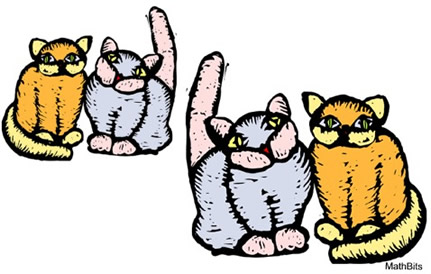How many transformations are needed to transform the cats on the left to the cats on the right?
Choose:
 1 2 3 4

9.
Given ΔABC with A(1,1), B(2,3) and C(3,1). If ΔA'B'C' is the result of a reflection in the y-axis, followed by a dilation of scale factor 2 centered in the origin, then ΔA'B'C' is ...
Choose:
 similar to ΔABC congruent to ΔABC neither similar nor congruent to ΔABC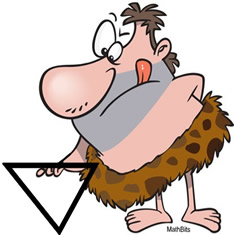10.

What are the coordinates of point A', the image
of point A(-4,1) after a reflection in the line
y = x, followed by a rotation of 90º centered
in the origin?

Choose:
 (-1,-4) (-4,-1) (1,4) (4,1)